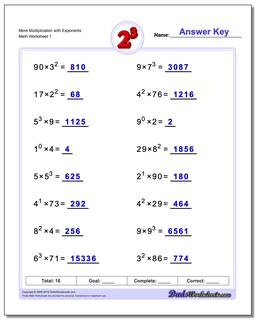# Math Worksheets: Exponents: Exponents: More Multiplication with Exponents## More Multiplication with Exponents

PropertyValue
DescriptionMore Multiplication with Exponents: Practice exponents worksheets mixing exponents with simple multiplication. Again, these are fantastic exercises for enhancing student comprehension of basic order of operations concepts where exponents are involved.
Resource TypeWorksheet